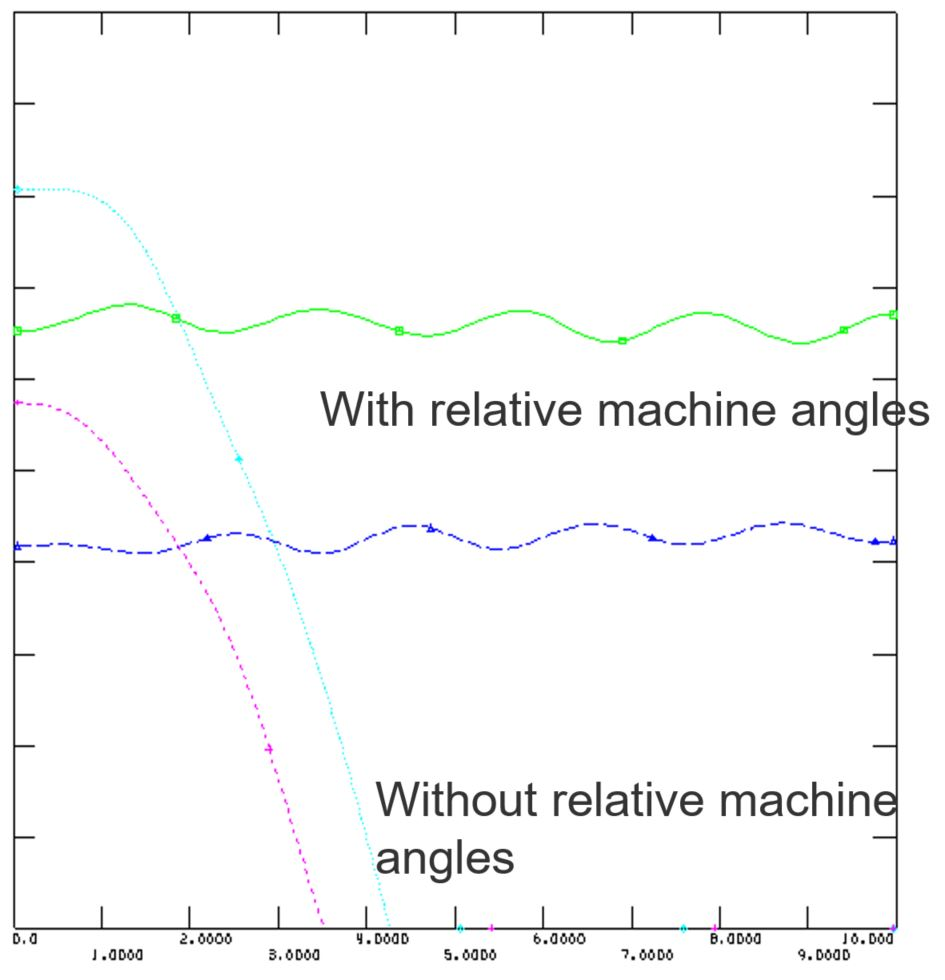# Network frequency dependence and relative machine angles in Dynamic Simulation Options

Hi,

I have two questions:

1. What happens during the dynamic simulation if you enable 'network frequency dependence' option?
2. Is it to necessary to set relative angles checked in the dynamic simulation? If yes, should it be set to the slack generator?

edit retag close merge delete

Sort by » oldest newest most votedNetwork frequency dependence option (model NETFRQ) is described in Program Application Guide, vol II, Chapter 25.3. The option should be activated whenever the frequency deviates from nominal frequency. I recommend to always use the option if the system is not very, very stiff with small deviations from nominal frequence.

Selection of relative angles do not change the results of the simulation, so it is not necessary. However the plotting of rotor angles channels are affected and it is often better to use relative angles when plotting simulations with under or over frequency. For a normal system it is a good rule to use "Weighted average angle" as system reference.

The plot below shows the rotor angles for two generators in a dynamic simulation. The green and blue curves are showing the result with weighted average angles while the other two curves shows the same generators when relative angles are not set in the simulation. There is a drop in absolute rotor angle due to underfrequency. The oscillation between the generators is clearly seen when using relative angles and can not be seen with absolute angles due tp the fast decrease of the absolute angle.more